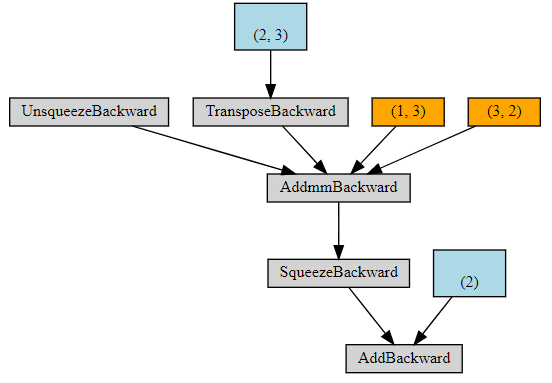# [SOLVED] Extra variables being added to computation graphs

Hi all,

While using the `visualize` tool of szagoruyko I have found that, for specific functions, there are more variables in the computation graph than those that I had defined. In particular, I’m referring to these two examples:

``````a = Variable(torch.rand(3))
b = nn.Parameter(torch.rand(2, 3))

y = b.mv(a)
make_dot(y)
``````

This one gives me this graphBlue rectangles represent parameters, orange ones represent variables, and grey ones are operations. Another simple example is the following:

``````c = nn.Parameter(torch.rand(2))
z = F.linear(a, b, c)
make_dot(z)
``````

Which gives me the following graphAs you might see, there are two variables, of sizes (2, 3) in the first example and (3, 2) in the second one, that I did not define but are nonetheless being used to perform the computations.

Does any one know what are these tensors? Can they alter the gradients that I compute in a backward pass?

If you look at the function in the first example, you can see that the `mv` operation is actually done in the backend with the more general `addmv` function (which adds a constant to the output). This is where this extra `(2,3)` Variable comes from.
In the second graph, you can see all the internals of how the `Linear` layer is implemented. Here the `addmm` functions is used, and so here again you see an extra `Variable` being used for the add part.